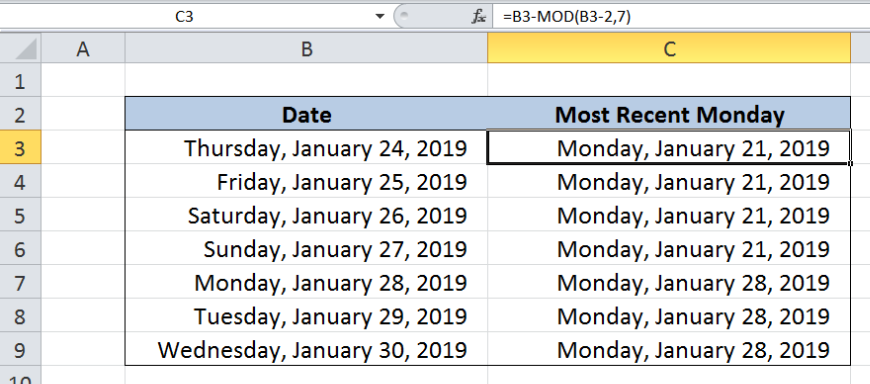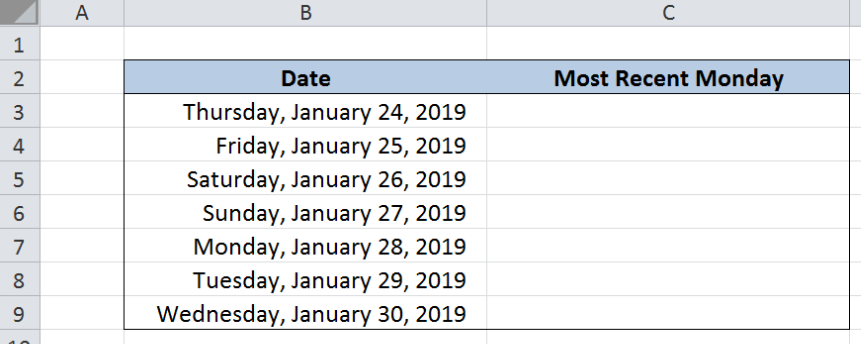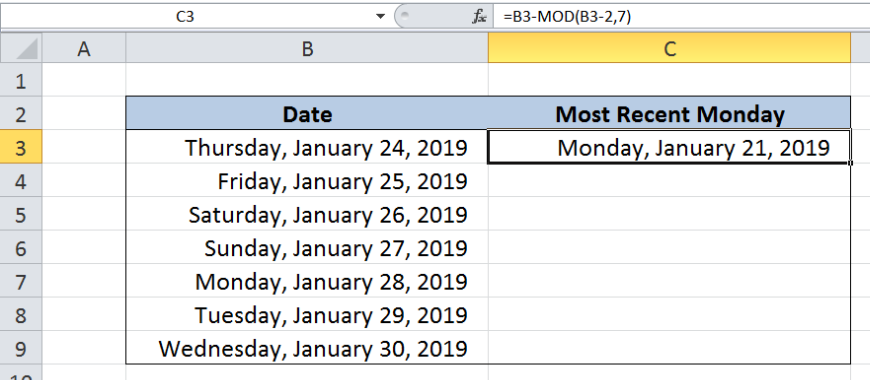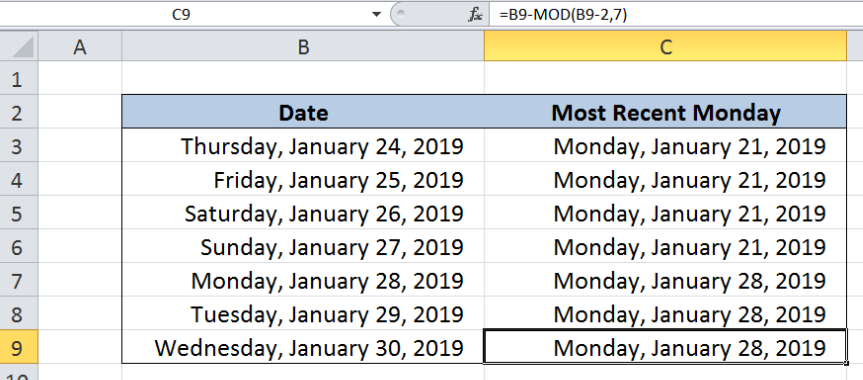Get instant live expert help with Excel or Google Sheets“My Excelchat expert helped me in less than 20 minutes, saving me what would have been 5 hours of work!”

#### Post your problem and you’ll get expert help in seconds.

Your message must be at least 40 characters
Our professional experts are available now. Your privacy is guaranteed.

# How to Get the Most Recent Day of the Week in Excel

In most calculations involving dates and day of the week, Excel provides a solution and saves us enormous amount of time from performing calculations on our own.  This step by step tutorial will assist all levels of Excel users in getting the most recent day of week using the MOD function.Figure 1.  Final result: Get most recent day of week

Final formula: `=B3-MOD(B3-2,7)`

## Syntax of MOD Function

MOD function returns the remainder after dividing a number by a divisor; the result of MOD always follows the sign of the divisor

`=MOD(number, divisor)`

• number  – the number for which we want to divide by a divisor and find the remainder
• divisor  – the number by which we want to divide number

## Syntax of TODAY Function

`=TODAY()`

• TODAY function does not have any arguments
• TODAY returns the current date
• It can be used in combination with other functions and mathematical operations to obtain the desired results

## Setting up our Data

Our table consists of two columns: Date (column B) and Most Recent Monday (column C).  We have a list of dates in column B, formatted such that the day of the week is displayed for easier reference.  We want to determine the most recent Monday and record the results in column C.Figure 2.  Sample data to get most recent day of week

By default, each day of the week is assigned a number by Excel where “1” refers to Sunday and “7” refers to Saturday.  For this example, we want to get the most recent Monday. Monday has the day of value of “2”.

## Get most recent Monday

In order to get the most recent Monday, we will be using a formula with the MOD function.  Let us follow these steps:

Step 1.  Select cell C3

Step 2.  Enter the formula: `=B3-MOD(B3-2,7)`

Step 3.  Press ENTER

Step 4.  Copy the formula in C3 to cells C4:C9 by clicking the “+” icon at the bottom-right corner of cell C3 and dragging it downFigure 3.  Get most recent Monday by using the MOD function

Our formula subtracts a value to the date in column B such that the result is the most recent Monday.  This part of the formula MOD(B3-2,7) subtracts the day of the week, in this case 2, from the date then divides the result by 7.  The remainder from the division operation is then subtracted from the date.

For our first example, B3 is a Thursday so its value is 5.  The formula then becomes:

` = B3-MOD(5-2,7)`

` = B3-MOD(3,7)`

` = B3-3`

The most recent Monday is three days before the date in B3, or three days before January 24, 2019.  Finally, our formula returns the date January 21, 2019, which is a Monday.

The table below shows the results for the most recent Monday in column C.  Note that for row 7, the date “January 28, 2019” is already a Monday. Hence, the date in C7 is the same date as in B7.Figure 4.  Output: Get most recent Monday

## Note

We can determine the most recent day of the week from any current date by using the TODAY and MOD function.  Let’s say we want to get the most recent Monday as of today, we use the formula:

`=TODAY()-MOD(TODAY()-2,7)`

If we want a different day of the week other than Monday, we simply replace the number “2” with the appropriate day of the week.

Most of the time, the problem you will need to solve will be more complex than a simple application of a formula or function. If you want to save hours of research and frustration, try our live Excelchat service! Our Excel Experts are available 24/7 to answer any Excel question you may have. We guarantee a connection within 30 seconds and a customized solution within 20 minutes.

### Did this post not answer your question? Get a solution from connecting with the expert.Another blog reader asked this question today on Excelchat:
Solution examplesI would need a formula what copies from my DataInput sheet into another one daily the first two column A and B.
Solved by K. S. in 33 minsI would need a formula what copies from my DataInput sheet into another one daily the first two column A and B.
Solved by B. C. in 60 minsI need the formula for time accrual to auto populate in E4 based on certain criteria. example: B4 = hire date E4= accrual rate If todays date is equal to or greater than 60 days but less than 1 year from B4 date then E4 = 1 If todays date is equal to or greater than 3 years but less than 4 years from B4 date then E4 = 1.08 If todays date is equal to or greater than 5 years but less than 9 years from B4 date then E4 = 1.5 If todays date is equal to or greater than 10 years but less than 14 years from B4 date then E4 = 1.58 If todays date is equal to or greater than 15 years from B4 date then E4 = 1.92
Solved by A. H. in 60 minsHello. I would like to create a drop down option to choose Square Feet or Square Meters. Next column they insert the amount and the next it converts the value to whatever is chosen from the drop down option. Can you help please?
Solved by Z. Q. in 39 minsHi there. I need a column to automatically calculate the numbers of days from a start time to today's date.
Solved by T. F. in 16 mins## Subscribe to Excelchat.coAnother blog reader asked this question today on Excelchat: